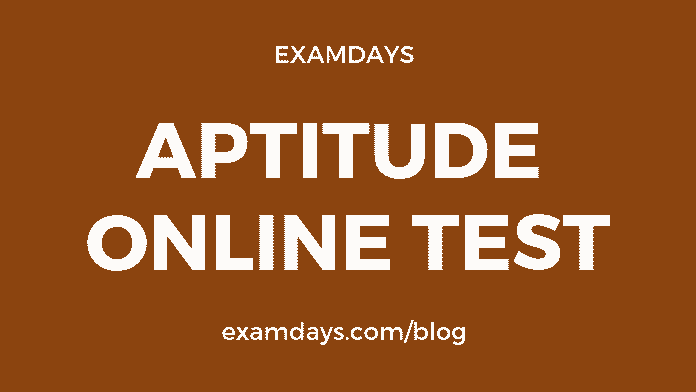Aptitude Online Test Quiz #7 with Answers

0Aptitude Online Test

General quantitative aptitude online test is more important for the various examinations, such as SSC, Railways RRB, PSU, State PSC, IBPS Banks, and other Government notifications, The aptitude questions practice make sense in the examination purpose, candidates should practice as many of questions and different models of questions.

Q1. What is the sum of this series 1 + 1 + 2 + 8 + 3+ 27 + 4 + 64 +_______10 + 1000?

1. 308
2. 3008
3. 3800
4. 3080

3080

Q2. There are 20 people in a party. If every person shakes hand with every other person, what is the total number of handshakes?

1. 150
2. 170
3. 190
4. 210

190

Q3. Let x, y and z be distinct integers. x and y are odd and positive, and z is even and positive. Which one of the following statements cannot be true?

1. (x – z)^2 y is even
2. (x – z) y^2 is odd
3. (x – z) y is odd
4. (x + y)^3 z is even

(x – z)^2 y is even

Q4. What is the value of M and N respectively? If M39048458N is divisible by 8 & 11; where M & N take integer values from 0 to 9, inclusive?

1. M = 7; N = 8
2. M = 8; N = 6
3. M = 4; N = 6
4. M = 6; N = 4

M = 6; N = 4

Q5. The Ratio’s Of Akash & Vinesh Age Is 9:7.If The Difference Between The Present Age Of Akash & The Age Of Vinesh 5 Years Hence Is 3.Then Find The Sum Of Their Present Ages?

1. 32
2. 64
3. 28
4. 42

64

Q6. If the ratio of ages of A and B is 7:2 while the sum of their ages is 45 years. What is the age of B?

1. 10 Years
2. 20 Years
3. 30 Years
4. 40 Years

10 Years

Q7. A Women’s Age Is 120% Of What It Was 15 Years Ago, But  75 % Of What It Will Be After 15 Years. What Is Her Present Age?

1. 32
2. 65
3. 37
4. 67

65

Q8. Find x and y so that the ordered data set has a mean of 42 and a median of 35.
17 , 22 , 26 , 29 , 34 , x , 42 , 67 , 70 , y

1. x = 32 , y = 66
2. x = 16 , y = 55
3. x = 36 , y = 77
4. x = 46 , y = 88

x = 36 , y = 77

Q9. If Arun’s birthday is on May 25 which is Monday and his sister’s birthday is on July 13. Which day of the week is his sister’s birthday?

1. Sunday
2. Saturday
3. Monday
4. Tuesday

Monday

Q10. At what time, between 3 o’clock and 4 o’clock, both the hour hand and minute hand coincide each other?

1. 3:16 4/11
2. 3:16 11/4
3. 3:16 7/11
4. 3:30